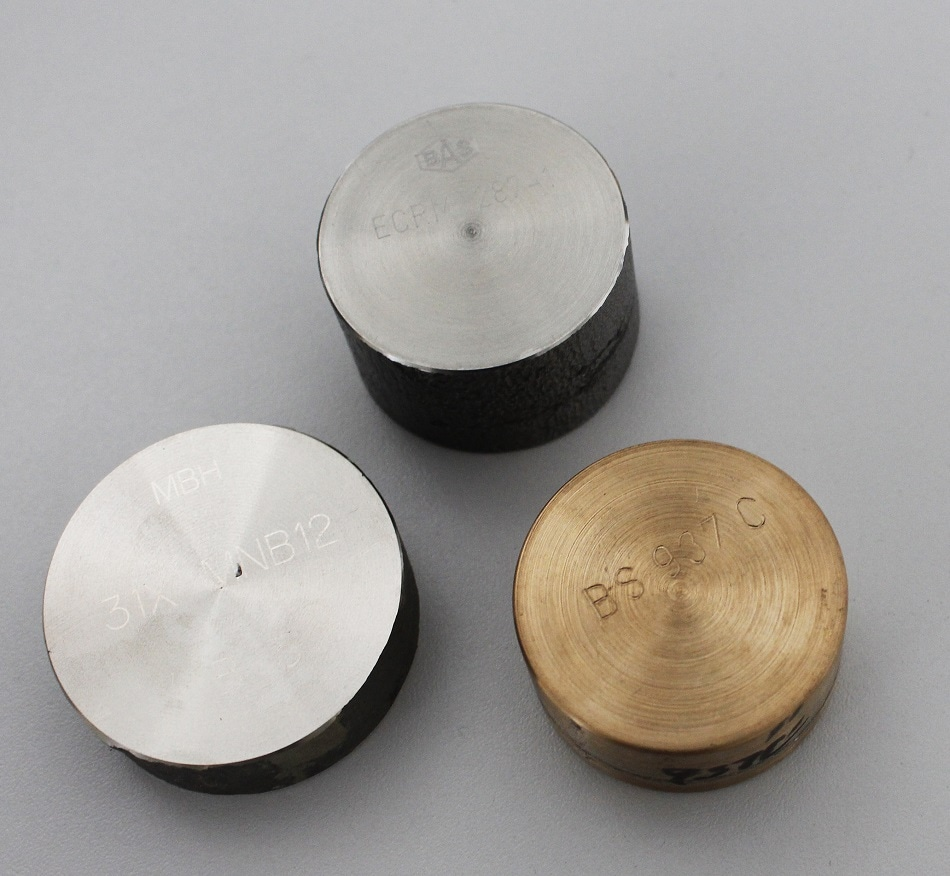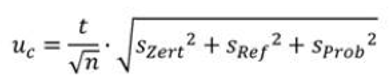# Using GUM to Calculate Measurement UncertaintyThe technique which is recommended by the regulatory bodies ISO (International Organisation for Standardisation) and BIPM (Bureau International des Poids et Mesures) is one of the most comprehensive techniques used to calculate measurement uncertainty. The technique is known as GUM, which stands for Guide to the Expression of Uncertainty in Measurement.

This technique is employed when you possess a reference sample of composition which is similar to what you expect in your unknown (production) sample, and that reference sample has a certified compositional value. The reason that this technique is so comprehensive is that it considers all error margins of every measurement gathered to obtain an accurate result.

So it includes, the error in your own measurements of the reference sample, the error of the certified value of the reference sample, and the error of your measurements of the unknown sample. According to GUM, the expression for calculating a confidence interval is:Before looking at an example, see each of the terms in this expression outlined below:

t is the value of the Student t-distribution function. Essentially, this is a multiplication factor utilized in any statistical system where the number of measurements (values) is relatively small. The value of t is dependent on two factors: the desired confidence interval and the number of measurements. The value for t can be acquired from published tables.

n is the number of measurements that have been gathered of your unknown sample and reference sample.

sprob is the standard deviation of the measurements of the unknown sample.

sref is the standard deviation of the measurements of the reference sample.

szert is the standard deviation of the certified value of the reference sample. This is gathered from the certificate which is supplied with the reference.

## Example of Utilizing GUM

The first step is to select a confidence level when calculating the measurement uncertainty. In this instance, 95% is used, as it is extremely common in spectroscopy.

The following values for the level of chromium in a sample are used:

Cr
Average value of reference sample from certificate 18.46%
Standard deviation of reference sample from certificate szert 0.05%
Average value of reference sample from analysis (10 readings) 18.54%
Standard deviation of reference sample from analysis sref 0.1%
Average value of unknown sample from analysis (10 readings) 18.82%
Standard deviation of unknown sample from analysis sprob 0.15%

t: 2.262 for a confidence level of 95% and sample size of 10
n: 10 (10 measurements of each sample)

Putting these figures into the above expression results in:

Uc = 0.134%.

So, according to GUM guidelines, it is 95% certain that the unknown sample has Cr at a composition of 18.82% +/- 0.13%.

Yet, the possible systematic deviation of the mean has not been considered, only the random fluctuations around the mean have been considered. This accuracy of the mean (or trueness) must be taken into account for a total value of measurement uncertainty.This information has been sourced, reviewed and adapted from materials provided by Hitachi High-Tech Analytical Science.

## Citations

• APA

Hitachi High-Tech Analytical Science. (2020, January 15). Using GUM to Calculate Measurement Uncertainty. AZoM. Retrieved on July 05, 2020 from https://www.azom.com/article.aspx?ArticleID=18464.

• MLA

Hitachi High-Tech Analytical Science. "Using GUM to Calculate Measurement Uncertainty". AZoM. 05 July 2020. <https://www.azom.com/article.aspx?ArticleID=18464>.

• Chicago

Hitachi High-Tech Analytical Science. "Using GUM to Calculate Measurement Uncertainty". AZoM. https://www.azom.com/article.aspx?ArticleID=18464. (accessed July 05, 2020).

• Harvard

Hitachi High-Tech Analytical Science. 2020. Using GUM to Calculate Measurement Uncertainty. AZoM, viewed 05 July 2020, https://www.azom.com/article.aspx?ArticleID=18464.